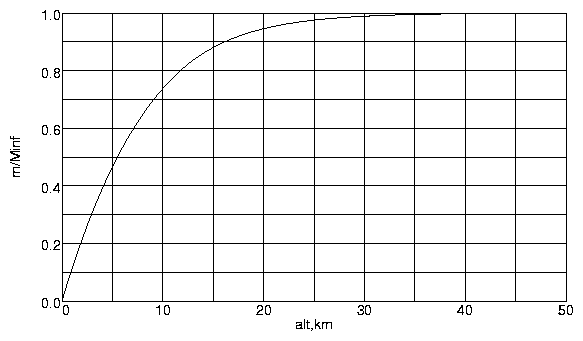PDAS Home > Contents > Difficult Problems > Atmosphere Thickness
Public Domain Aeronautical Software (PDAS)

This is not a good question because it does not have a precise answer. The atmosphere gets less dense as you go up in altitude, but it never quite goes to zero. Similar questions are: "How long does it take for a capacitor to discharge?" or "How long does it take for radioactive material to decay?". These are all examples of asymptotic behavior. But, much in the same way that we make a definition that allows us to speak of the thickness of a boundary layer in viscous fluid flow, we can give a definition of the thickness of the atmosphere that satisfies our engineering needs.

To make this estimation, the first thing to do is to compute the mass distribution of the atmosphere. For a given altitude, define m(alt) to be the total mass of air from sea level up to alt over the entire earth. This is simply a matter of integrating the density of the atmosphere over the volume between a sphere of radius E and a sphere of radius alt+E, where E is the radius of the earth. You may examine my page that describes the numerical details of performing this numerical integration.
XHTML+MathML, 5KB or PDF, 44KB.

The results of this computation are shown in the table and chart below. Note that m is given in zettagrams. One zettagram = 10**21 grams = 1 Zg. Zetta is the prefix for 10**21, just as we use peta for 10**15 and tera for 10**12, and Z stands for zetta when combining with an abbreviated unit such as g for gram.

alt (km)m (Zg)m/
m(infinity)
000
21.1350.2147
52.4610.4655
103.8990.7376
154.6530.8801
204.9990.9455
255.1560.9752
305.2280.9888
355.2610.9950
405.2760.9979
455.2830.9993
505.2871.0000Using boundary layer theory as an example, we can define the thickness of the atmosphere to be the altitude that encloses 99 percent of the total mass of the atmosphere. Looking at the chart, we can see that this seems to be about 31 kilometers. The halfway point, where half the mass of the atmosphere is below and half above occurs at 5.5 kilometers. Another interesting fact is that when you are cruising in a modern jet transport at 11 kilometers, you are above 77.5 percent of the atmosphere. The total mass of the atmosphere turns out to be 5.3 zettagrams (5.3 Zg).

If we say that the thickness of the earth's atmosphere is 31km, we are making a definition based on enclosing 99 percent of its mass. But this is an arbitrary choice. If we define the thickness as enclosing 99.9 percent of the mass, the answer is 42km.

Another method for establishing this value is to compare the orbits of objects entering the earth's atmosphere, either natural or man-made. At high altitudes, the tracks are indistinguishable from Keplerian orbits for a body in vacuum. Below a certain height, the paths differ from the vacuum orbits. This height seems to be around 75 kilometers. When the X-prize people were making the rules for the contest for putting a manned craft into space, they rounded it to 100 km. If you demand that the particle count per cubic meter be indistinguishable from the density of the solar wind in the vicinity of the earth's orbit, you have to go to something like 1000 km. Certainly it is apparent that the thickness of the atmosphere is quite small compared to the diameter of the earth. If you have seen my page on the subject Why doesn't the atmosphere fall to the ground?, you may agree with me that the atmosphere has already fallen to the surface of the earth. It can't get any closer. It is only because we are so small in the scale of the universe that we perceive the atmosphere to be immense. It is tiny and fragile.

If you find zettagrams, petabytes and femtoseconds a bit challenging, have a look at the NIST prefix page.

PDAS Home > Contents > Difficult Problems > Atmosphere Thickness
Public Domain Aeronautical Software (PDAS)